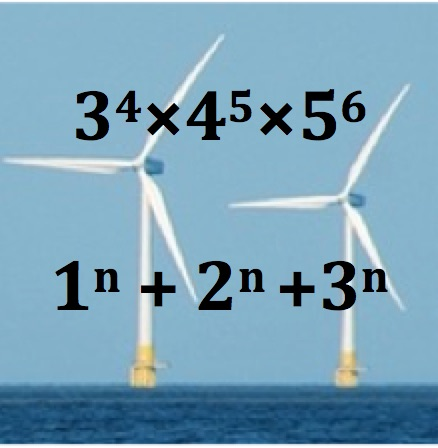1.   Imagine the first number $$3^4\times 4^5\times 5^6$$ is written out in full. How many zeros would there be at the end? Why? Can you find the answer without doing the whole calculation?

2.   Work out a few values of the second number $$1^n+2^n+3^n$$ with n = 1, 2, 3 and 4 . Can you show numbers like this will be even for all values of n?

For what values of n will $$1^n+2^n+3^n+4^n$$ be even.

What about $$1^n+2^n+3^n+4^n + 5^n$$?

What can you say about the sums of powers of the counting numbers 1, 2, 3, 4, 5, 6, 7, …p?

Tagged with: